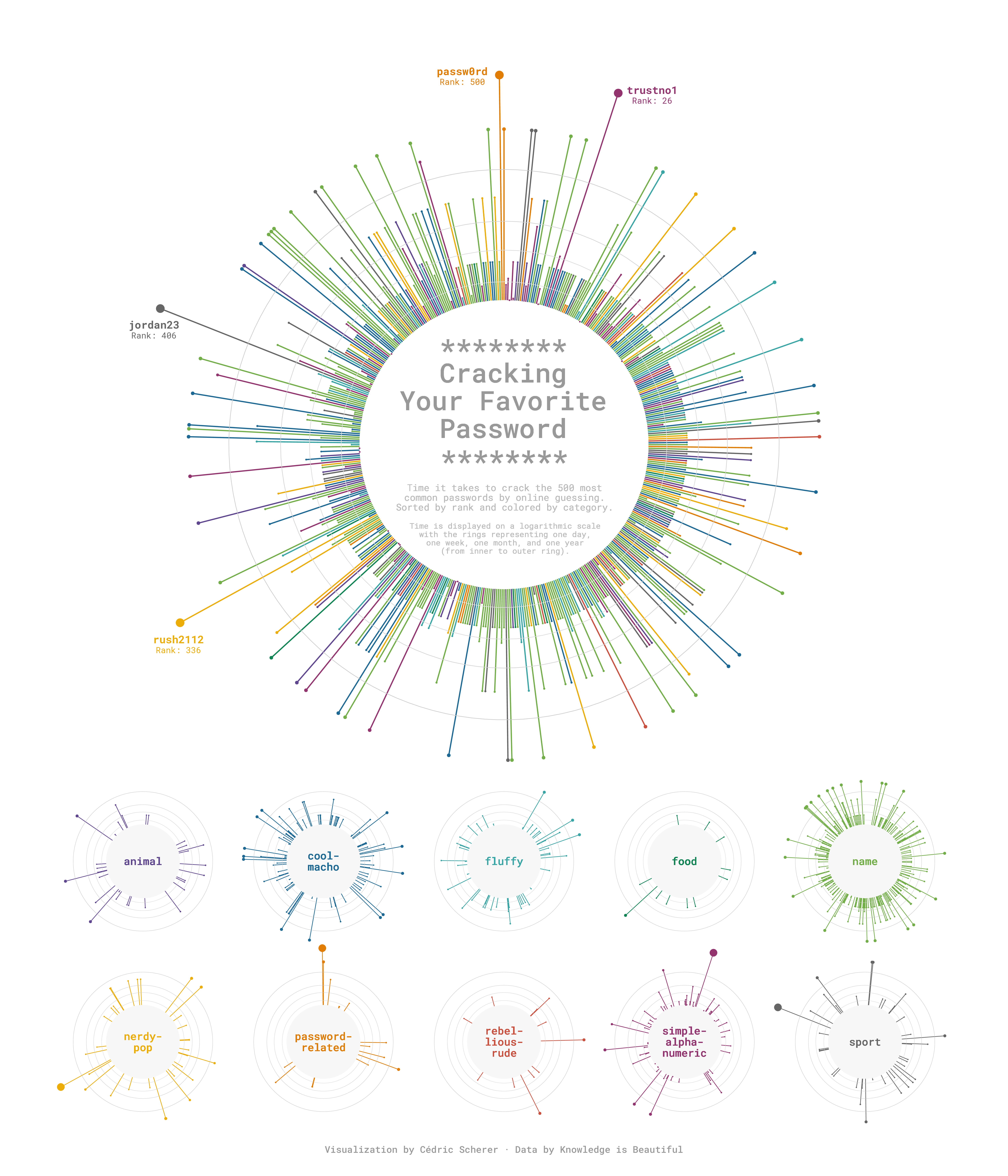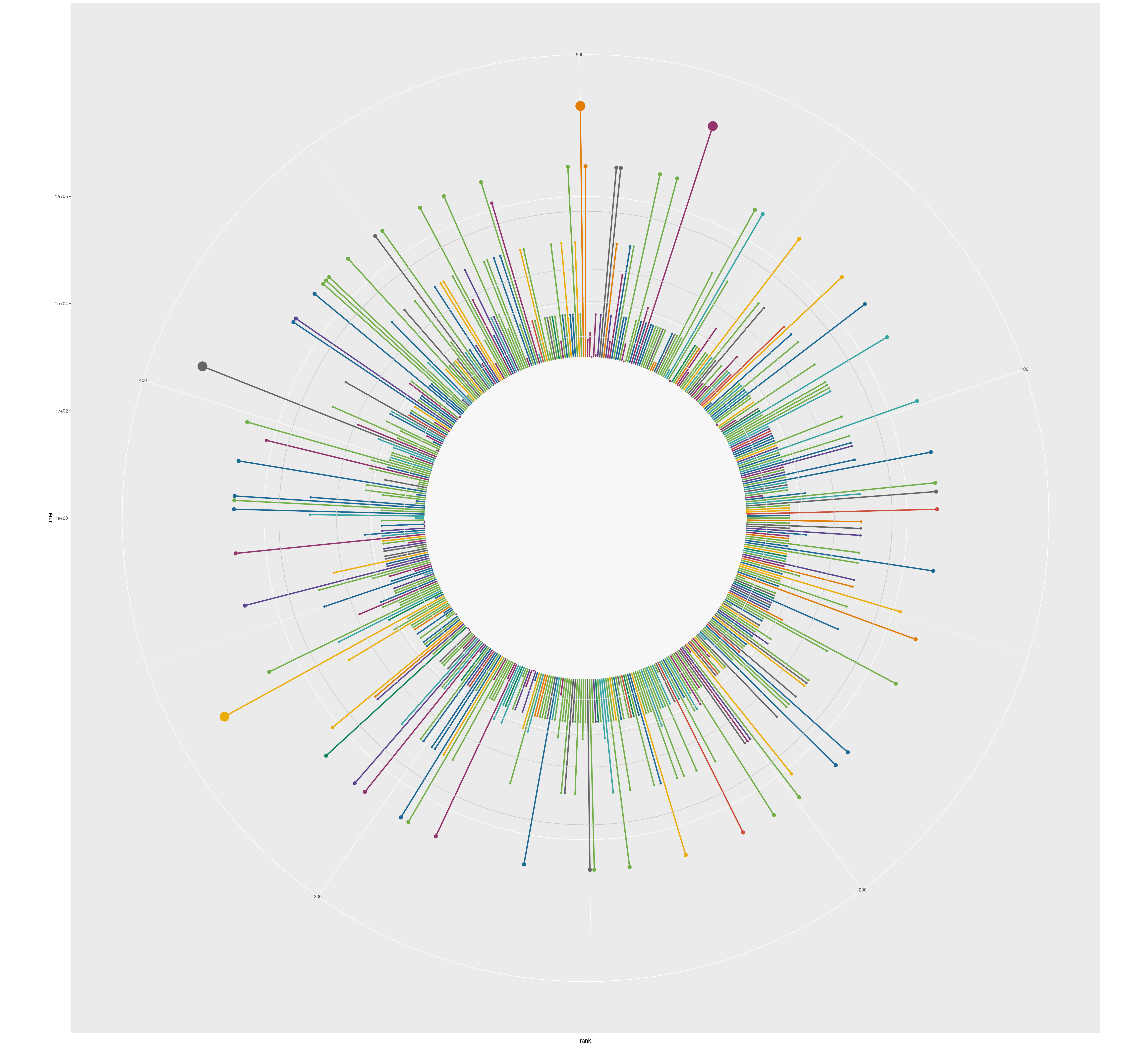# Circular lollipop plot with R and ggplot2

A circular lollipop plot with a highly customized layout to explore password popularity and strengths made with `R` and `ggplot2`. This blogpost guides you through a step-by-step construction of a custom circular lollipop plot that includes a variety of tricks to make the final result much more beautiful.

This page showcases the work of Cédric Scherer, built for the TidyTuesday initiative. You can find the original code on his Github repository here.

Thanks to him for accepting sharing his work here! Thanks also to Tomás Capretto who split the original code into this step-by-step guide! 🙏🙏

As a teaser, here is the plot we’re gonna try building:Let’s start by loading the packages needed to build the figure. `ggtext`, and `patchwork` stand out today. `ggtext` makes it very easy to style text with Markdown or HTML, and `patchwork` allows us to compose ggplot2 figures very smoothly.

``````library(tidyverse)
library(ggtext)
library(patchwork)``````

Today, the plot is going to be based on the Roboto Mono font family. If you are unsure about how to work with custom fonts in `R`, this post is for you.

# Load and prepare the data

This guide shows how to create a beautiful multipanel circular lollipop plot to visualize password popularity and strengths.

The data for this post comes from Information is Beautiful. This guide uses the dataset released for the TidyTuesday initiative on the week of 2020-01-14. You can find the original announcement and more information about the data here. Thank you all for making this guide possible!

``````df_pw <- readr::read_csv('https://raw.githubusercontent.com/rfordatascience/tidytuesday/master/data/2020/2020-01-14/passwords.csv') %>%
# Remove rows where the password is missing

From all the columns in the data frame, only `rank`, `password`, `category`, `value`, and `time_unit` are going to be used. Let’s see what they mean:

• `rank` Popularity in the database of released passwords
• `password` The text of the password
• `category`The category where the password falls into
• `value` Time to crack by online guessing
• `time_unit` Time unit to match with value

The next step is to convert all the time values to a common unit such as seconds. This task suits very well for the `case_when()` function.

``````df_pw_time <-
df_pw %>%
mutate(
time = case_when(
time_unit == "seconds" ~ value,
time_unit == "minutes" ~ value * 60,
time_unit == "hours" ~ value * 60 * 60,
time_unit == "days" ~ value * 60 * 24,
time_unit == "weeks" ~ value * 60 * 24 * 7,
time_unit == "months" ~ value * 60 * 24 * 30,
time_unit == "years" ~ value * 60 * 24 * 365,
TRUE ~ NA_real_
)
) ``````

Next, a fixed value of 1000 is added to all the times. This is going to leave the extra space needed for the labels within the circle.

``````plus <- 1000
df_pw_plot <-
df_pw_time %>%
mutate(time = time + plus) %>%
add_row(rank = 501, time = 1)``````

And finally, create a data frame with all the information required to place labels for passwords that were really hard to crack.

``````labels <-
df_pw_plot %>%
# Value > 90 selects those above 90 years!
filter(value > 90) %>%
# Create label out of the password and its rank
mutate(label = glue::glue("<b>{password}</b><br><span style='font-size:18pt'>Rank: {rank}</span>")) %>%
# These columns give the position of the labels
x = c(33, 332, 401, 492),
y = c(75000000, 90000000, 45000000, 48498112)
)``````

Did you note that HTML within the label string? That is one of the many ways to tell `ggtext` how to customize the text in the plot. That library is great!

# Basic circular plot

Let’s start by creating the circular plot on the top panel:

``````main <- ggplot(df_pw_plot, aes(rank, time, color = category)) +
# This segment represents the vertical lines
geom_segment(
aes(x = rank, xend = rank, y = 0, yend = time),
size = 1.2
) +
# This rect is converted into the inner circle where we're going to place text
# when converting the plot to circular coordinates.
geom_rect(
aes(xmin = 1, xmax = 501, ymin = 0, ymax = plus),
fill = "grey97", color = "grey97"
) +

# These lines indicate one day, one week, one month and one year.
geom_hline(aes(yintercept = (1 * 24 * 60 + plus)), color = "grey88") +
geom_hline(aes(yintercept = (7 * 24 * 60 + plus)), color = "grey85") +
geom_hline(aes(yintercept = (30 * 24 * 60 + plus)), color = "grey82") +
geom_hline(aes(yintercept = (365 * 24 * 60 + plus)), color = "grey79") +

# Add points on the end of each line
geom_point(aes(size = time)) +

# Polar axis (y-axis) is in log10 scale
scale_y_log10(expand = c(0, 0)) +

# Use the Prism color scale for the categories
rcartocolor::scale_color_carto_d(palette = "Prism", guide = "none") +

# Assign a range for the size of the dots
scale_size(
range = c(1, 8),
limits = c(plus, max(df_pw_plot\$time)),
guide = "none"
) +

# Make it circular!
coord_polar()

main``````Converting a plot from cartesian to circular (polar) coordinates in `ggplot2` is as easy as adding a `coord_polar()` call to the plot. So neat!

The plot obtained so far looks quite nice, but it’s not done. It still lacks something that indicates how to read the story this chart is trying to tell and the labels that highlight those very hard to crack passwords. Let’s do it!

``````main <- main +
geom_richtext(
data = labels,
aes(x = x, y = y, label = label, color = category),
lineheight = 0.8,
size = 8,
family = "Roboto Mono",
label.color = NA
) +
# Regular text with `geom_text()`
# Note that coordinates are explicitly passed here.
geom_text(
x = 500, y = 1.2,
family = "Roboto Mono Medium",
size = 20,
lineheight = 0.87,
color = "grey60"
) +
geom_text(
x = 250, y = 0.25,
label = "********",
family = "Roboto Mono Medium",
size = 20,
lineheight = 0.87,
color = "grey60"
) +
geom_text(
x = 250, y = 1.1,
label = "Time it takes to crack the 500 most\ncommon passwords by online guessing.\nSorted by rank and colored by category.",
family = "Roboto Mono",
size = 7,
lineheight = 0.87,
color = "grey73"
) +
geom_text(
x = 250, y = 1.95,
label = "Time is displayed on a logarithmic scale\nwith the rings representing one day,\none week, one month, and one year\n(from inner to outer ring).",
family = "Roboto Mono",
size = 6,
lineheight = 0.87,
color = "grey73"
)

main```````geom_richtext()` is kind of magical. Just give it got some text formatted with HTML and it takes care of everything needed to succesfully protray the formatted label in the figure.

So far, this is enough for the top-panel figure. Further modifications such as a different background color and margin adjustments are going to happen when this plot is composed with the lower-panel figures.

# Multi panel plot

The original plot we’re trying to replicate consists of several panels and all the work above only replicates the bigger panel on top. The good news is that `ggplot2` makes it really easy to reuse existing code for a plot and convert it into a plot made of multiple panels.

First of all, it’s necessary to add line breaks to some existing category names so they fit within the inner circle.

``````facet_data <-
df_pw_plot %>%
add_row(rank = 501, time = 1, category = unique(df_pw_plot\$category)) %>%
# This is where we add line breaks
mutate(
cat_label = case_when(
category == "cool-macho" ~ "cool-\nmacho",
category == "nerdy-pop" ~ "nerdy-\npop",
category == "rebellious-rude" ~ "rebel-\nlious-\nrude",
category == "simple-alphanumeric" ~ "simple-\nalpha-\nnumeric",
TRUE ~ category
)
) %>%
filter(!is.na(category))``````

And now let’s make the plot!

``````# Much of the code is the same than the code above.

facet <- ggplot(facet_data, aes(rank, time, color = category)) +
geom_segment(
aes(x = rank, xend = rank, y = 0, yend = time),
size = 0.6
) +
geom_rect(
aes(xmin = 1, xmax = 501, ymin = 0, ymax = plus),
fill = "grey97", color = "grey97"
) +
geom_hline(aes(yintercept = (1 * 24 * 60 + plus)), color = "grey82", size = 0.2) +
geom_hline(aes(yintercept = (7 * 24 * 60 + plus)), color = "grey79", size = 0.2) +
geom_hline(aes(yintercept = (30 * 24 * 60 + plus)), color = "grey76", size = 0.2) +
geom_hline(aes(yintercept = (365 * 24 * 60 + plus)), color = "grey73", size = 0.2) +
geom_point(aes(size = time)) +

# This is what adds the label within each circle
geom_text(
aes(label = cat_label, color = category),
x = 500, y = 0,
family = "Roboto Mono Medium",
size = 8,
lineheight = 0.87
) +
# This is what wraps the panels for the categories into a layout of 2 rows
facet_wrap(~ category, nrow = 2) +
coord_polar() +
scale_y_log10(expand = c(0, 0)) +
rcartocolor::scale_color_carto_d(palette = "Prism", guide = "none") +
scale_size(
range = c(0.5, 7),
limits = c(plus, max(df_pw_plot\$time)),
guide = "none"
) +
theme(
strip.text = element_blank(),
)

facet``````Did you spot the difference in the code? Just adding `facet_wrap(~ category, nrow = 2)` tells `ggplot2` to create a plot for each level in the `category` variable and arrange them in a 2-row layout.

# Final chart

The two plots obtained above can be composed very easily into a single figure thanks to the `patchwork` package. At this stage, it is also important to adjust the margins and the space between the panels to obtain a better appearece. Are you ready to finish? Let’s go!

``````# Customize the main panel (top-panel)
main <- main +
# This 'theme_void' adds a completely empty theme
theme_void() +
# These negative margins are VERY important to obtain a tight layout.
theme(
plot.margin = margin(-50, -180, -70, -180, "lines"),
)

facet <- facet +
# Same theme_void for the lower panels
theme_void() +
theme(
# Negative spacing between panels, which means they overlap
panel.spacing = unit(-8, "lines"),
# Negative margin on top, to decrease blank space between these panels
# and the main plot on top
plot.margin = margin(-40, 50, 10, 50)
) +
# Adjust settings for the caption
theme(
plot.caption = element_text(
family = "Roboto Mono Medium",
size = 20,
color = "grey60",
hjust = 0.5,
margin = margin(-50, 10, 30, 10)
)
) +
labs(caption = "Visualization by Cédric Scherer ∙ Data by Knowledge is Beautiful")

# Combine the two plots
# The '+' means to 'add' the two ggplot2 plots together.
panel <- (main + facet) +
plot_layout(
ncol = 1, # Results in one plot on top and another on the bottom.
heights = c(1, 0.28) # Height assigned to each plot
)
panel``````Awesome, we nailed it!!🥳

Related chart types

## Contact

This document is a work by Yan Holtz. Any feedback is highly encouraged. You can fill an issue on Github, drop me a message on Twitter, or send an email pasting yan.holtz.data with gmail.com.